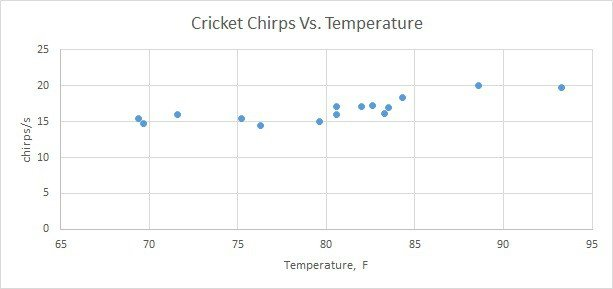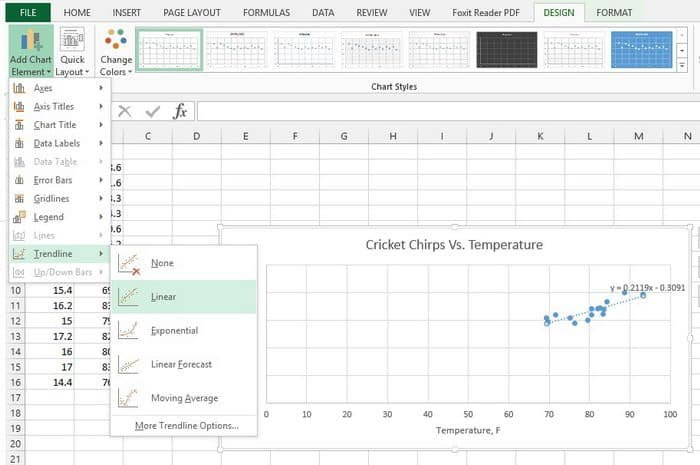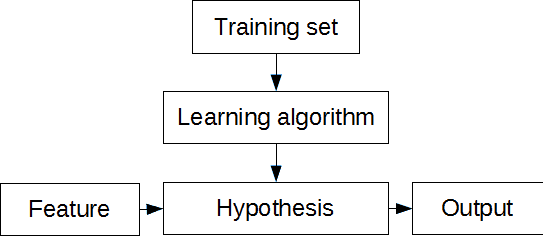# Simplest machine learning algorithm – linear regression with excel

Some may say that linear regression is more statistical problem. And this is truth at some level. But when problem is solved from machine learning perspective, things gets easier especially when moving towards more complex problems.

First of all lets understand few important terms. We can start with regression. When speaking of linear regression we try to find best fitting line through given points. In other words we need to find optimal linear equation to fit given data points. This is a supervised learning problem when we have set of data pairs that can be plot on x-y axis. I understand, theory is boring thing, even for me, so lets move to practical example and learn by solving some problem.

In order to work with some examples we need sample data. There are many data sources available on internet. For instance great source is college cengage that have several sets with data pairs meant for linear regression problems. For our example we are going to use Cricket Chirps Vs. Temperature data where each data point consists of chirps/sec and temperature in degrees Fahrenheit. You can send data in three formats: excel, mtp and ascii. Lets download excel data where we can immediately draw graph and see what’s going on.Right away in excel, we can additionally do some analysis. For this we need to click on the excel chart then go to appeared DESIGN tab and select Add Chart Element and select Trend Line -> Linear.Excel automatically adds trend line which is nothing more than linear regression. You can select Format Trendline where you can check Display Equation on chart check box. As you can see for our data set linear regression formula is

y = 0.2119x-0.3091

What does this mean practically. We have learned two linear formula coefficients 0.2119 and 0.3091 that can be used to predict cricket chirp frequency at any given temperature. For instance we can take any temperature from the range and even out of it and calculate the chirp/sec value. As example lets take temperature 85F according to formula we get

y = 0.2119 · 85 – 0.3091 = 17.7Hz.

Another example. We can try to speculate with values out of range. For instance we would like to know the chirp frequency when temperature is 50F. Again lets apply our learned formula and calculate:

y = 0.2119 · 50 – 0.3091 = 10.29Hz

So we have a simple machine which can approximately predict values on given parameter.

In this example temperature values we call features and chirp/sec values – target variables or outputs. Continuing with machine learning lexicon the data set we used for training linear regression parameters is called training set. The formula we used for trend line is called hypothesis. The only thing missing here is learning algorithm.In excel this process is hidden but is suitable for simple problems like this. And as you already guessed, you can use different types of trend lines (logarithmic, exponential, polynomial, power, etc.) that fit training set best. Using excel is easy with one variable (univariate) data sets, but when working with multidimensional data things get more complicated. Next time we are going to focus on learning algorithm for univariate data set and then move on to more complex (multivariate) data sets and hypotheses.